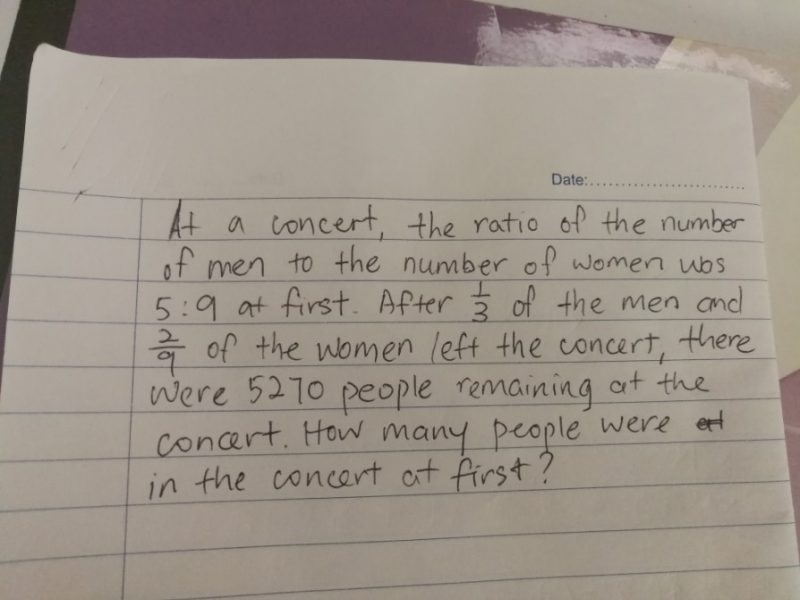# QuestionHi

Need help to solve the question.

Thanks

Initial ratio

Men : Women

5       :  9

x 3

15     : 27

1/3 of men leave, so 5U of men leave, remaining is 10U

2/9 of women leave, so (2/9) x 27 = 6U leave, remaining is 21U

Total units remaining = 10 + 21 = 31U.

31U = 5270, 1U = 170.

At start there were 15 + 27 = 42U. 42 x 170 = 7140

0 Replies 1 Like